# What is a Plus Perfect Number and How to Calculate It in Fortran 90

Knoji reviews products and up-and-coming brands we think you'll love. In certain cases, we may receive a commission from brands mentioned in our guides. Learn more.
Plus Perfect Numbers are a class of very interesting numbers in the â€˜Recreational Number Theoryâ€™. They are also called narcissistic numbers or Armstrong numbers. Plus perfect number is the number that is equal to the sum of its digits each ra

Number Theory is one of the most beautiful branches of mathematics. A class of very interesting numbers in the ‘Recreational Number Theory’ is the Plus Perfect Numbers. In this article I am going to discuss what a plus perfect number is and how to compute such numbers.

What is A Plus Perfect Number?

Plus perfect number is the number that is equal to the sum of its digits each raised to the power of the length of the number.They are also called narcissistic numbers or Armstrong numbers.

The definition becomes little bit clearer with the help of an example. Let us start with the number 1634. Length of it is 4 and (1^4)+(6^4)+(3^4)+(4^4)=1634. That is why 1634 is a plus perfect number. All the 1-digit numbers are Armstrong numbers. After 9, the lowest more than 1-digit Armstrong number is 153. Few more examples are 370, 371, 407, 8208, 9474 etc.

Base Dependence:

One interesting thing about Plus perfect numbers is that they are base dependent. For example 99 is not plus perfect in 10 base but it is in 6 base. As 99=(2*6^2)+(4*6^1)+(3*6^0), 99 in 10 base equals to 243 in 6 base and (2^3)+(4^3)+(3^3)= 99 =(243)_6 base.

But here we will work with 10-base numbers only.

How to check and compute plus perfect numbers?

We can check if a given number is plus perfect or not and calculate those kind of numbers in a certain range using any programming language but in this article I will use Fortran 90 which is an easy and popular language.

For this we need to know the algorithm of separating the digits of the number which is actually very easy. It follows some steps:

1) First we have to divide the number by 10. The remainder is the last digit of the number. ­­

2) The integer quotient of the first step will be the dividend for the next step.

3) Then again we have to divide the dividend by 10 to get the 2nd last digit of the number and calculate the remainder too.

4) We have to repeat this process until the quotient becomes zero for the next step.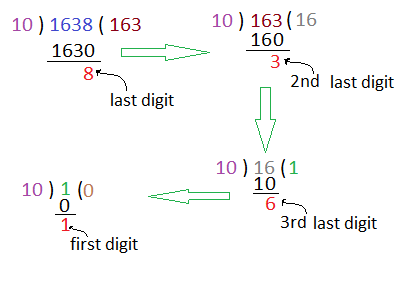Counting these digits we get the length of the number.

Rest is trivial. We have to raise the digits to the power of the length of the number, add them up and check if the number is equal to the sum calculated just now or not.

The Fortran 90 Programming:

Examples of calculating narcissistic numbers in Fortran 90 are given below.

i)Let us start with identifying any given number to be plus perfect number or not.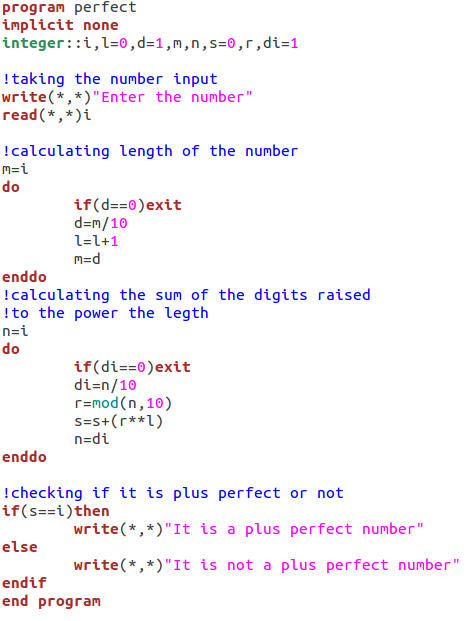ii)We can do this job by using functions and subroutines too. The program is given here.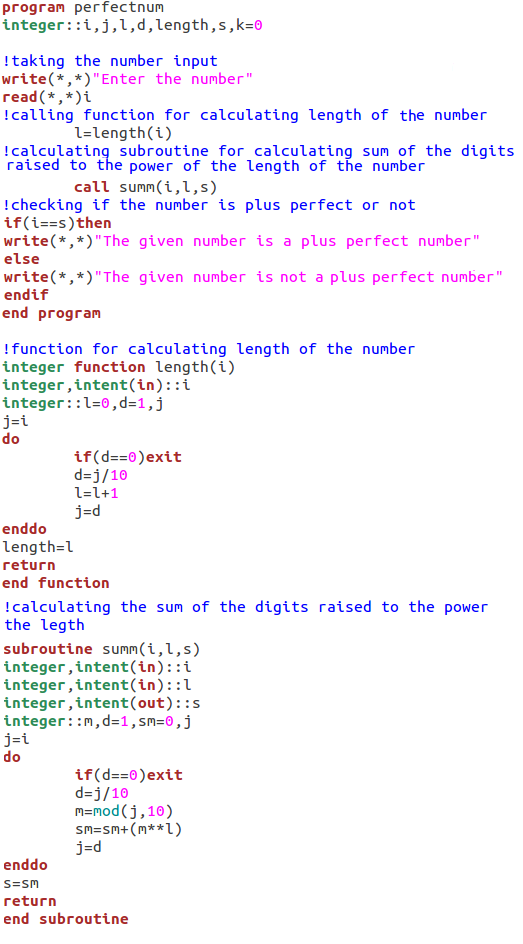iii) Now we can proceed to calculate all the narcissistic numbers in a certain given range, count how many are they and we can set the range according to user’s wish.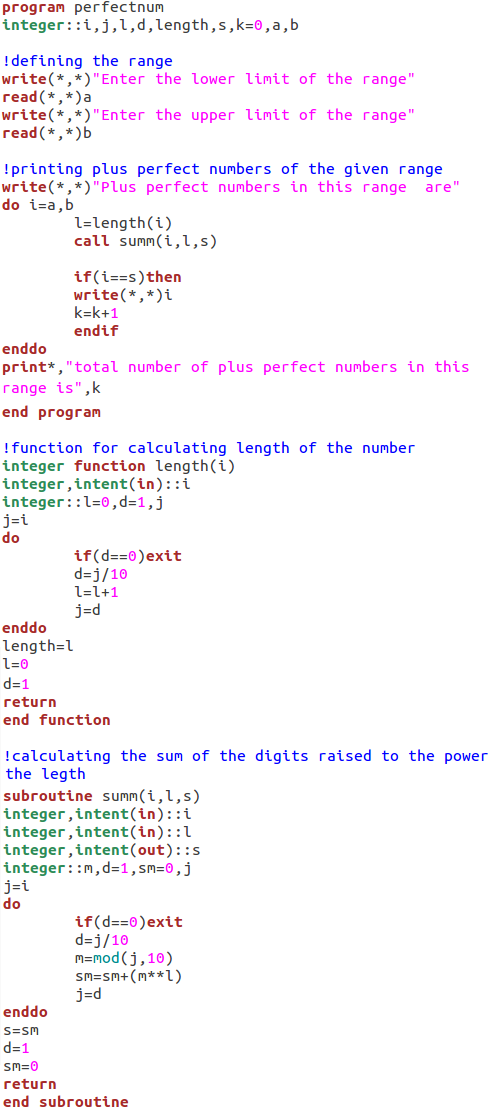So we can see that Armstrong numbers or plus perfect numbers can be calculated very easily in Fortran 90.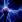Sonia
0
Posted on May 5, 2012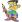roytanay
0
Posted on May 5, 2012